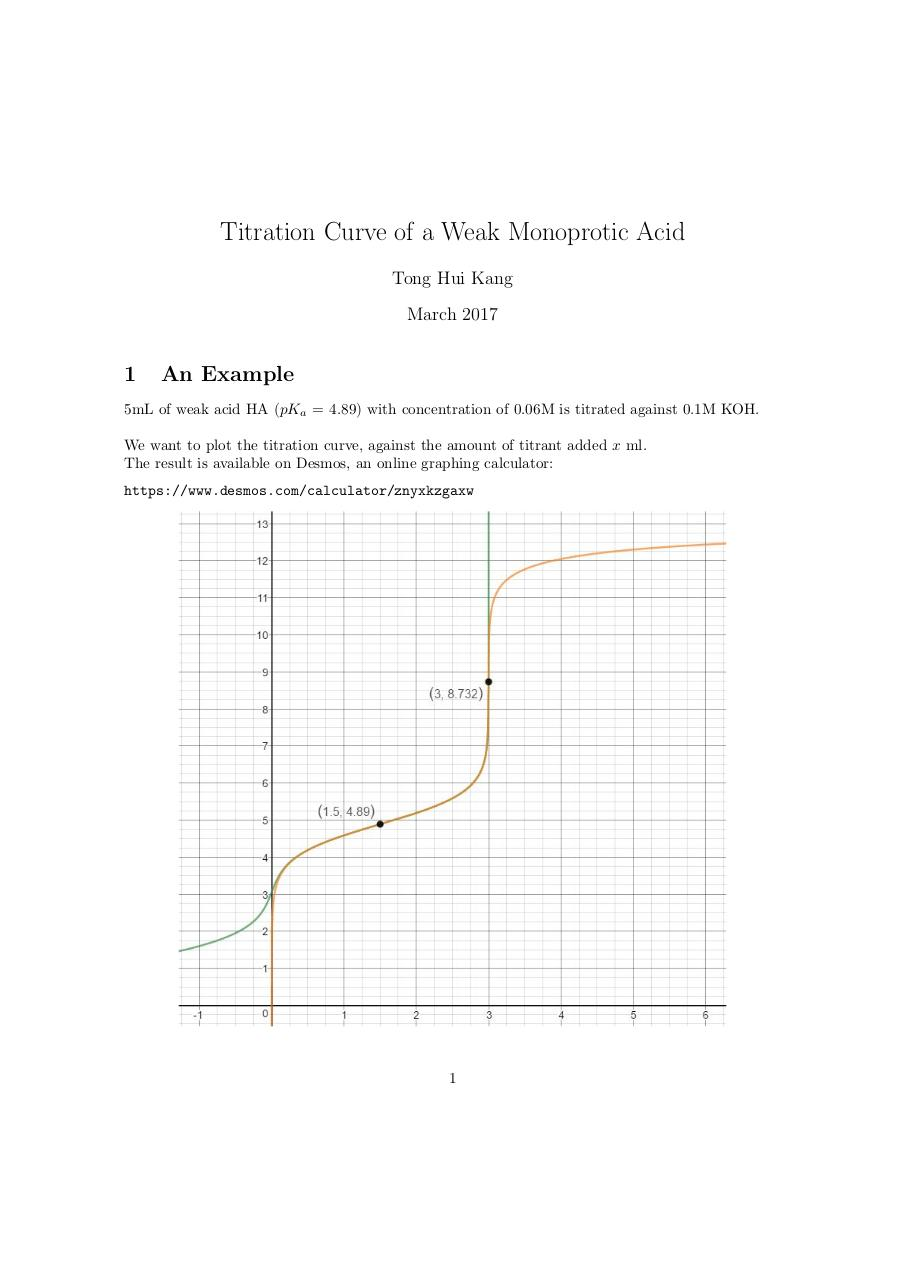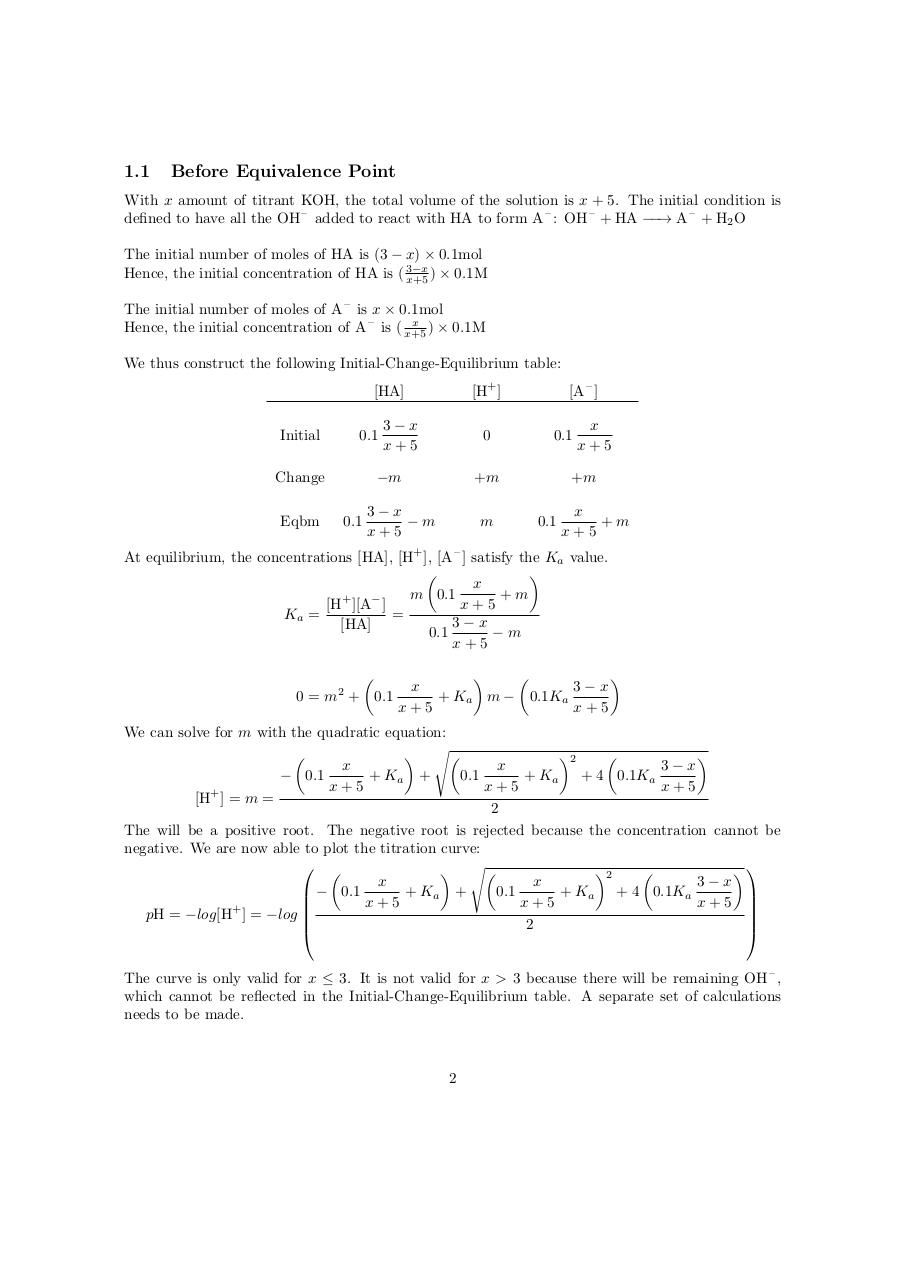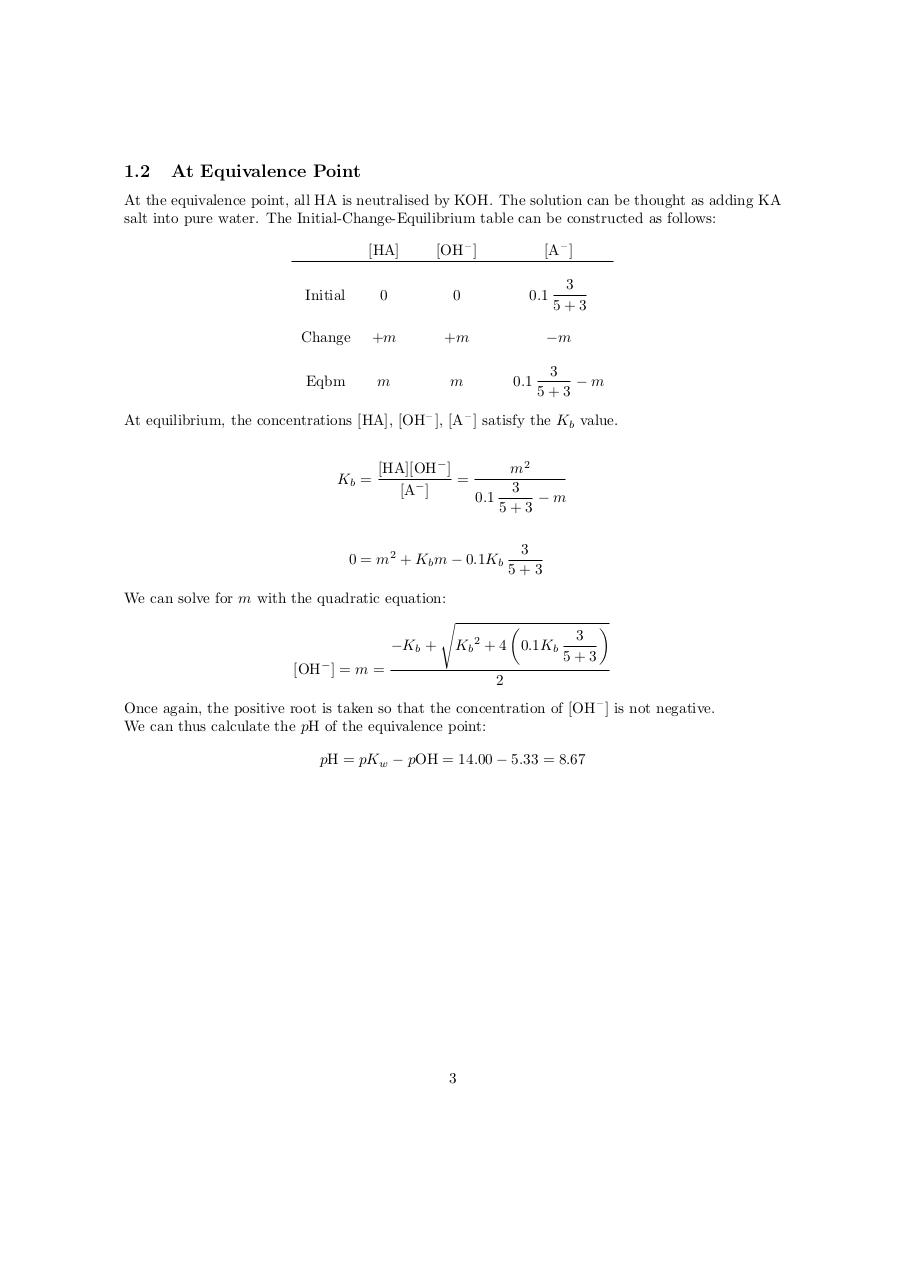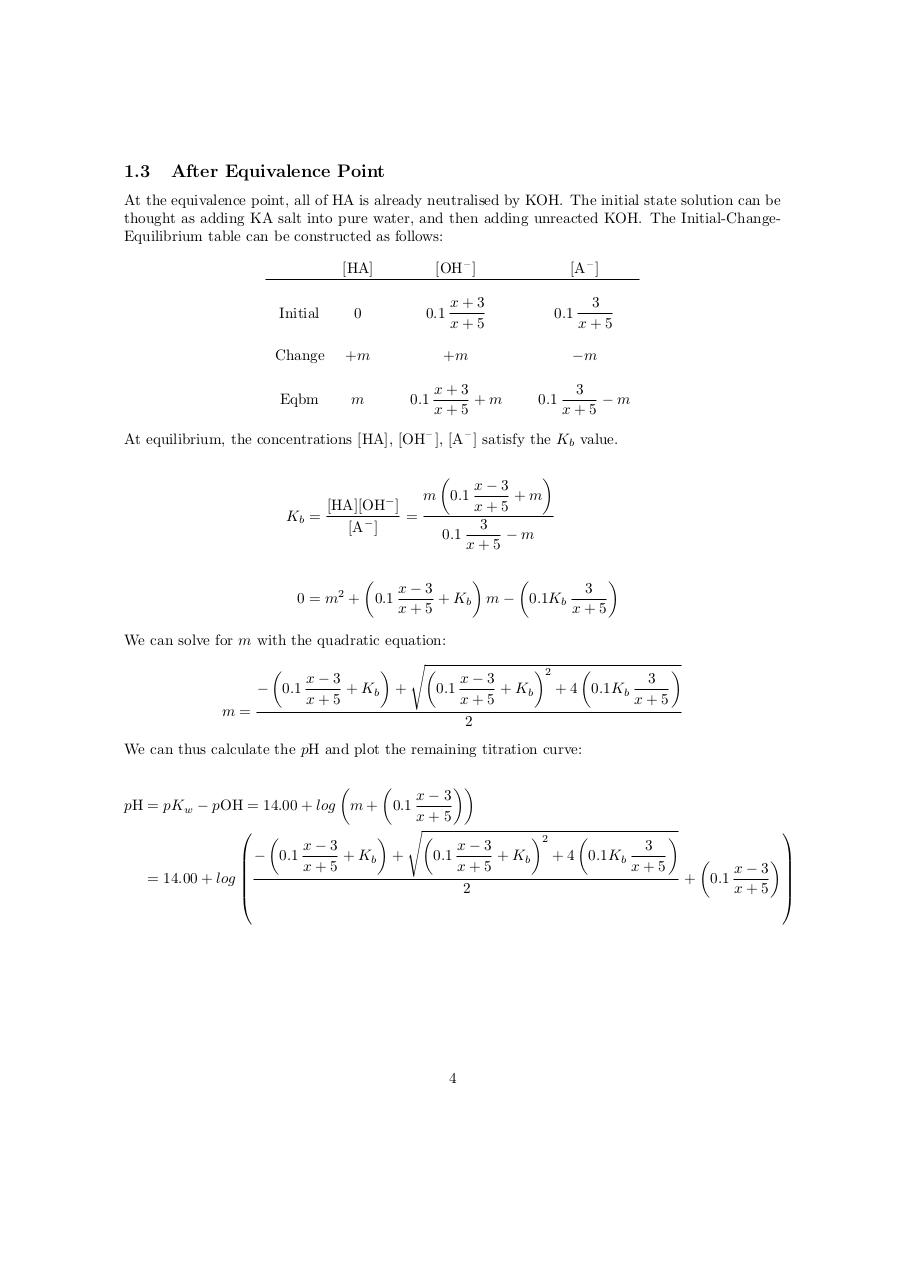# Titration Curve (1) .pdf

### File information

Original filename: Titration_Curve (1).pdf

This PDF 1.5 document has been generated by TeX / pdfTeX-1.40.17, and has been sent on pdf-archive.com on 19/03/2017 at 15:41, from IP address 138.75.x.x. The current document download page has been viewed 1053 times.
File size: 211 KB (4 pages).
Privacy: public file

Titration_Curve (1).pdf (PDF, 211 KB)

### Document preview

Titration Curve of a Weak Monoprotic Acid
Tong Hui Kang
March 2017

1

An Example

5mL of weak acid HA (pKa = 4.89) with concentration of 0.06M is titrated against 0.1M KOH.
We want to plot the titration curve, against the amount of titrant added x ml.
The result is available on Desmos, an online graphing calculator:
https://www.desmos.com/calculator/znyxkzgaxw

1

1.1

Before Equivalence Point

With x amount of titrant KOH, the total volume of the solution is x + 5. The initial condition is
defined to have all the OH – added to react with HA to form A – : OH – + HA −−→ A – + H2 O
The initial number of moles of HA is (3 − x) × 0.1mol
Hence, the initial concentration of HA is ( 3−x
x+5 ) × 0.1M
The initial number of moles of A – is x × 0.1mol
x
) × 0.1M
Hence, the initial concentration of A – is ( x+5
We thus construct the following Initial-Change-Equilibrium table:
[H+ ]

[HA]
Initial

0.1

0

−m

Change
Eqbm

3−x
x+5

0.1

[A – ]
0.1

+m

3−x
−m
x+5

m

x
x+5

+m
0.1

x
+m
x+5

At equilibrium, the concentrations [HA], [H+ ], [A – ] satisfy the Ka value.


x
m 0.1
+m
[H+ ][A− ]
x+5
Ka =
=
3−x
[HA]
0.1
−m
x+5

0 = m + 0.1
2




x
3−x
+ Ka m − 0.1Ka
x+5
x+5

We can solve for m with the quadratic equation:

 s
2


x
x
3−x
− 0.1
+ Ka +
0.1
+ Ka + 4 0.1Ka
x+5
x+5
x+5
+
[H ] = m =
2
The will be a positive root. The negative root is rejected because the concentration cannot be
negative. We are now able to plot the titration curve:
 
 s
2


x
x
3−x
+ Ka +
0.1
+ Ka + 4 0.1Ka
 − 0.1

x+5
x+5
x+5 
+

pH = −log[H ] = −log 

2

The curve is only valid for x ≤ 3. It is not valid for x &gt; 3 because there will be remaining OH – ,
which cannot be reflected in the Initial-Change-Equilibrium table. A separate set of calculations
needs to be made.

2

1.2

At Equivalence Point

At the equivalence point, all HA is neutralised by KOH. The solution can be thought as adding KA
salt into pure water. The Initial-Change-Equilibrium table can be constructed as follows:
[HA]

[OH – ]

Initial

0

0

Change

+m

+m

Eqbm

m

m

[A – ]
0.1

3
5+3

−m
0.1

3
−m
5+3

At equilibrium, the concentrations [HA], [OH – ], [A – ] satisfy the Kb value.

Kb =

[HA][OH− ]
=
[A− ]
0.1

m2
3
−m
5+3

0 = m2 + Kb m − 0.1Kb
We can solve for m with the quadratic equation:
s
−Kb +

3
5+3


Kb 2 + 4 0.1Kb

[OH− ] = m =

3
5+3



2

Once again, the positive root is taken so that the concentration of [OH – ] is not negative.
We can thus calculate the pH of the equivalence point:
pH = pKw − pOH = 14.00 − 5.33 = 8.67

3

1.3

After Equivalence Point

At the equivalence point, all of HA is already neutralised by KOH. The initial state solution can be
thought as adding KA salt into pure water, and then adding unreacted KOH. The Initial-ChangeEquilibrium table can be constructed as follows:
[OH – ]

[HA]
Initial

0

Change

+m

Eqbm

m

0.1

[A – ]

x+3
x+5

0.1

−m

+m
0.1

x+3
+m
x+5

3
x+5

0.1

3
−m
x+5

At equilibrium, the concentrations [HA], [OH – ], [A – ] satisfy the Kb value.



x−3
m 0.1
+m
[HA][OH− ]
x+5
Kb =
=
3
[A− ]
−m
0.1
x+5




x−3
3
0 = m + 0.1
+ Kb m − 0.1Kb
x+5
x+5
2

We can solve for m with the quadratic equation:

 s
2


x−3
x−3
3
− 0.1
+ Kb +
0.1
+ Kb + 4 0.1Kb
x+5
x+5
x+5
m=
2
We can thus calculate the pH and plot the remaining titration curve:



x−3
pH = pKw − pOH = 14.00 + log m + 0.1
x+5
 

 s
2


x−3
x−3
3
+ Kb +
0.1
+ Kb + 4 0.1Kb
 − 0.1



x+5
x+5
x+5
x−3 

= 14.00 + log 
+ 0.1
2
x+5 

4Use the permanent link to the download page to share your document on Facebook, Twitter, LinkedIn, or directly with a contact by e-Mail, Messenger, Whatsapp, Line..

Use the short link to share your document on Twitter or by text message (SMS)

#### HTML Code

Copy the following HTML code to share your document on a Website or Blog

#### QR Code### Related keywords